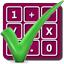#### Arithmetic of Computers

#### from Tenscope Limited

By using this site, you are accepting "session" cookies, as set out in the site's Privacy Policy
Cookies are also used to remember which page of the book you last viewed, so that when you revisit the site you automatically return to the last page you visited.

### Self-Test Questions : Lesson 1

#### The Powers of Numbers

##### A little exponent goes a long way!

1.
Are 100.00 and .03333 both decimal numbers?

2.
Are 9 and 6 factors of 15?

3.
How many factors can be multiplied together?

4.
In what order should factors be multiplied?

5.
What is ten used as a factor twice?

6.
If you use 4 as a factor 3 times, what is the result?

7.
What is the shorthand way for writing “60 used as a factor 100 times”?

8.
What is a power?

9.
How do you write “the third power of eight”?

10.
What are the names of the two numbers in the expression 63?

11.
What do we mean when we say “b” is 4 and “n” is 2?

12.
Does b1 equal “b” or “1”?

When you have finished, turn to Page 41 for Lesson 2.